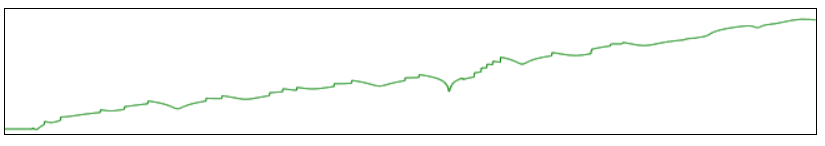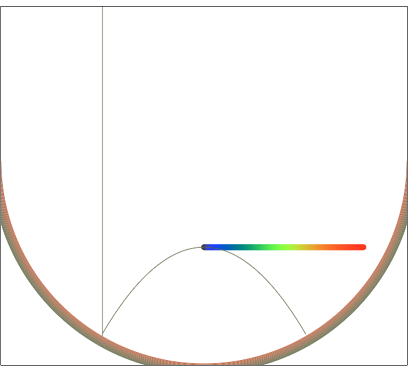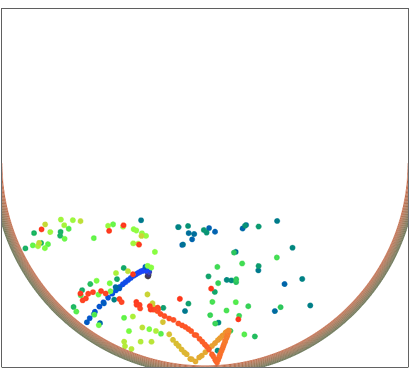# The onset of Chaos. Bouncing balls.

Up a level : Fractals and Chaos
Previous page : A family of fractals , the mod-curves
Next page : On the Bouncing balls programThe program on this page is demonstrating the onset of chaos. It is basically demonstrating the famous butterfly effect, where a small difference in the initial conditions many cause large changes after not all to long time.

A number of balls are dropped over a semi-circular bowl. The balls are initially separated by a selectable horizontal distance. The balls bounce with total elastic bounces from the bowl and from the walls. Since the slope of the bowl is different at different points along the bowl, ball with even the slightest offset will bounce in slightly different directions. As you will see when trying it, even the smallest offset will lead to wildly different paths of the balls. There are 256 balls, all with different colours.

The buttons

The program can be controlled with the mouse, touch or keys.• Wait/Start/Pause/Restart: (Esc or space ) Will start the program, pause it (and the timer stops too), and restart it. It will show the text Wait and be disabled for a second or so in the beginning. This during the time the program figures out the frame rate.
• Reset: (R) This will reset the positions to the initial positions, reset all variables and stop the program.
• Step: (S)This will advance the balls corresponding to a 4 ms actual runtime.
• Save: (D) Saves the Distance data to the clipboard. If you paste this into an Excel-file it will be placed in a column. More on this further down the page.
• Centre/Left: (C) Toggles between centrering the balls above the centre line of the bowl and placing them at the starting point to the left.
• Best: (B) This toggles between a couple of interesting cases. In the first it allows you to examine some very stable paths. Some, or all, of the balls will move close to the shown parabolic path. You can select the endpoint by dragging the vertical line. More on this further down the page. The second case is another two bounce case, where you may also drag the gray starting line. The third case is when the balls starts very close to the edge, causing them to get to chaos in a surprisingly short time
• Images/Drawn: (I) Selects if the balls are drawn circular disks or images.
• Trace On/Trace 1/Trace2/Trace Off  (T) Shows a trace after ball 0 or shows the path ball 0 will take.

Below the buttons you can select the initial horizontal separation of the balls. This is in pixels, assuming the ball has a radius of eight pixels. The smallest separation is 10-13 of a pixel. Smaller than this would surpass the 64 bit resolution of the numbers. Even at this separation some balls will actually have the same positions. The first setting spreads the balls evenly across above the bowl.

A distance of 10-9 pixels would on my screen that is 27 cm wide hand has a horizontal resolution of 1920 pixels  give a distance of
0.27/(1929⋅109)≈1.5⋅10-13  m,  or about 1/1000th of the size of an atom.

The balls have an initial position, but you may select another position by clicking on a place of your choice. If you have a touch screen you may also touch the screen at the point where you want the balls to start. You can then drag to get set the velocity.

The separation graph

Beneath the graph there is a smaller graph presenting the mean separation of initially adjacent balls. It plots time vs the log of the mean separation. As you will see it is somewhat linear, indicating that the separation grows exponentially over time. I have not added any scales to the graph since it is mainly used to give some general feeling for the change in distances. If you want to analyse it further, you can press the Save button. The separation is calculated as the average of the distances between ball 0 and 1, then 1 and 2, and so on.The time at which the balls are separated by a rather arbitrary value calculated from the height and width of the possible positions, are shown as the time of Chaos. The save button saves tab separated columns of data. The sample time (the distance data sampled every 10th ms), the average distance between successive balls at the corresponding time, the times when ball 0 will bounce off of something, and the corresponding times. This data can directly be pasted into an Excel file for further analysis.

The “best” graph

You can move around the graph by dragging the vertical grey line. The parabola shows the possible stable path. It has the property of being perpendicular to the semi-circular bowl at the points where the balls would bounce, and, with the right speed, it will just bounce right back.If you select the separation 10^0=1 pixel, then Best and Start you will see two stable paths emerge. One, corresponding to the blue set of balls, moving as indicated by the initial parabola, and one, corresponding to the redish balls,  by repeated bounces through the centre of the bowl. We have in this case two possible so-called attractors.You can do something similar in the second case. For the third case, select a very small distance between the balls.

The loops

The main program consists of ta loop in a loop. The outer loop updates the screen. It is a requestAnimationFrame loop. The inner loop updates the positions of the balls. It runs as many 0.1 ms steps as fit within one frame time.

The position of a ball is not calculated using some Euler step method or something similar. Instead, it is calculated using the equation of the parabolic path of the ball, The possible collisions with the walls are calculated, and if it is above the bowl, that collision is used. It is found by simply finding the intersection of the parabolic trajectory of the ball and respective wall If the collision is below the bowl, we need to find the actual collision with the bowl.  This is calculated solving the quartic (fourth degree) equation one can get by the system of equation given by the time dependent equation of the parabolic trajectory of a ball and the equation of the bowl. The first positive solution is then used. The function for the direction of the ball after the collision was found using some fairly straight forward vector algebra.  More on this here.

The time for the next collision is calculated directly at a given collision. This is then used to check when time has advanced enough for the next step.

The balls as images are sprites taken from a larger image, balls.pgn. I wrote another small program to draw the balls, using radialGradiens -among other things.Up a level : Fractals and Chaos
Previous page : A family of fractals , the mod-curves
Next page : On the Bouncing balls programLast modified: Aug 14, 2023 @ 21:12